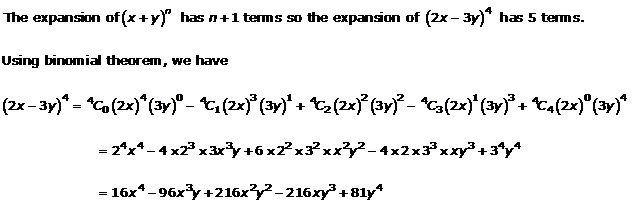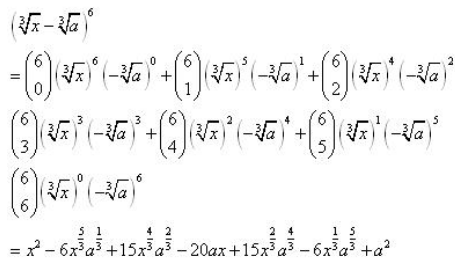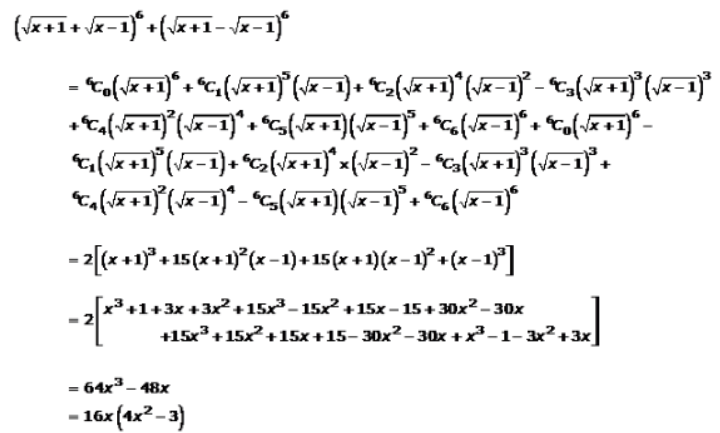×#### Thank you for registering.

One of our academic counsellors will contact you within 1 working day.

Click to Chat

1800-1023-196

+91-120-4616500

CART 0

• 0

MY CART (5)

Use Coupon: CART20 and get 20% off on all online Study Material

ITEM
DETAILS
MRP
DISCOUNT
FINAL PRICE
Total Price: Rs.

There are no items in this cart.
Continue Shopping• Complete JEE Main/Advanced Course and Test Series
• OFFERED PRICE: Rs. 15,900
• View Details

```Chapter 18: Binomial Theorem – Exercise 18.1

Binomial Theorem – Exercise – 18.1 – Q.1(i)Binomial Theorem – Exercise – 18.1 – Q.1(ii)Binomial Theorem – Exercise – 18.1 – Q.1(iii)Binomial Theorem – Exercise – 18.1 – Q.1(iv)Binomial Theorem – Exercise – 18.1 – Q.1(v)Binomial Theorem – Exercise – 18.1 – Q.1(vi)Binomial Theorem – Exercise – 18.1 – Q.1(vii)Binomial Theorem – Exercise – 18.1 – Q.1(viii)Binomial Theorem – Exercise – 18.1 – Q.1(ix)Binomial Theorem – Exercise – 18.1 – Q.1(x)Binomial Theorem – Exercise – 18.1 – Q.2(i)```### Course Features

• 728 Video Lectures
• Revision Notes
• Previous Year Papers
• Mind Map
• Study Planner
• NCERT Solutions
• Discussion Forum
• Test paper with Video Solution